# PHYS 205 Multiple Star Systems PHYS 205 Binary

• Slides: 9PHYS 205 Multiple Star Systems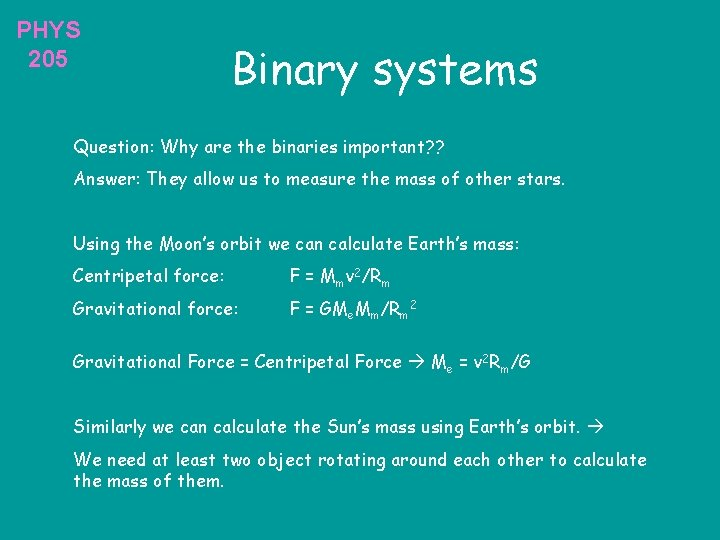PHYS 205 Binary systems Question: Why are the binaries important? ? Answer: They allow us to measure the mass of other stars. Using the Moon’s orbit we can calculate Earth’s mass: Centripetal force: F = Mmv 2/Rm Gravitational force: F = GMe. Mm/Rm 2 Gravitational Force = Centripetal Force Me = v 2 Rm/G Similarly we can calculate the Sun’s mass using Earth’s orbit. We need at least two object rotating around each other to calculate the mass of them.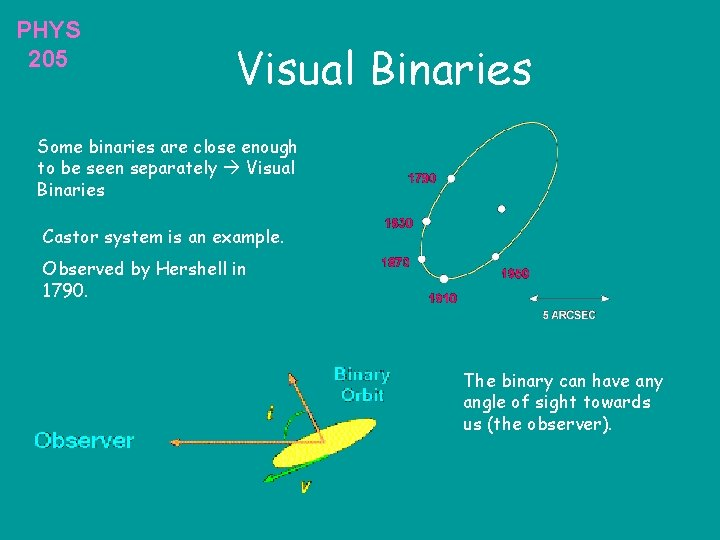PHYS 205 Visual Binaries Some binaries are close enough to be seen separately Visual Binaries Castor system is an example. Observed by Hershell in 1790. The binary can have any angle of sight towards us (the observer).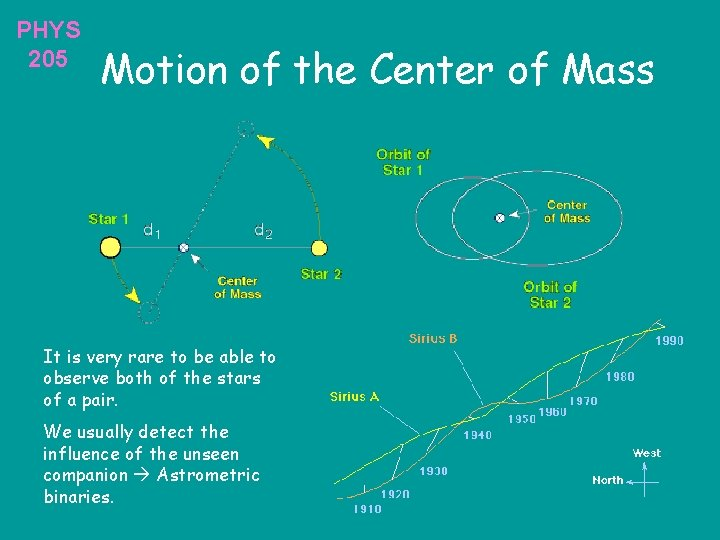PHYS 205 Motion of the Center of Mass It is very rare to be able to observe both of the stars of a pair. We usually detect the influence of the unseen companion Astrometric binaries.PHYS 205 Spectroscopic Binaries If the plane of rotation of the binaries is parallel to our line of sight, we might not see them, but their light will give us enough info about their nature.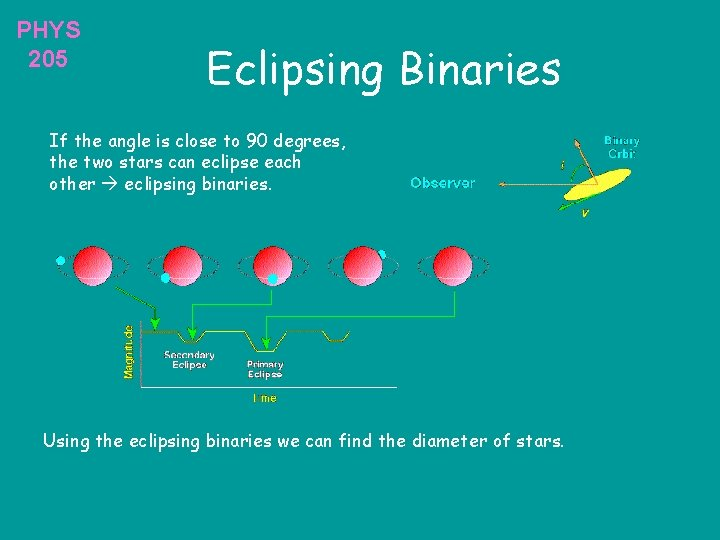PHYS 205 Eclipsing Binaries If the angle is close to 90 degrees, the two stars can eclipse each other eclipsing binaries. Using the eclipsing binaries we can find the diameter of stars.PHYS 205 Measuring the Diameter From the shape of the light curve and the duration of the eclipse we can calculate the diameter of the larger star. Not only binaries, but also planet systems are discovered this way. We can also use the Stefan-Boltzmann law L = 4 R 2 T 4. From the amount of light we are receiving, we measure the luminosity, and from the spectral analysis, we measure the color of the star and determine its temperature we can calculate the diameter indirectly.PHYS 205 Algol Binary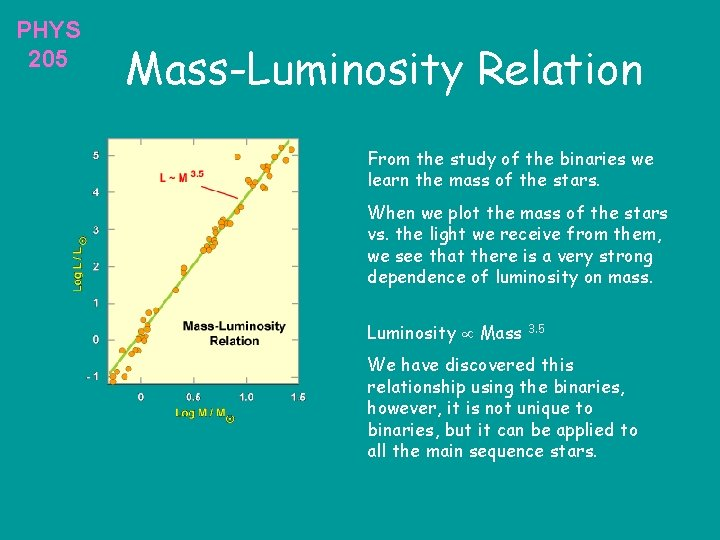PHYS 205 Mass-Luminosity Relation From the study of the binaries we learn the mass of the stars. When we plot the mass of the stars vs. the light we receive from them, we see that there is a very strong dependence of luminosity on mass. Luminosity Mass 3. 5 We have discovered this relationship using the binaries, however, it is not unique to binaries, but it can be applied to all the main sequence stars.